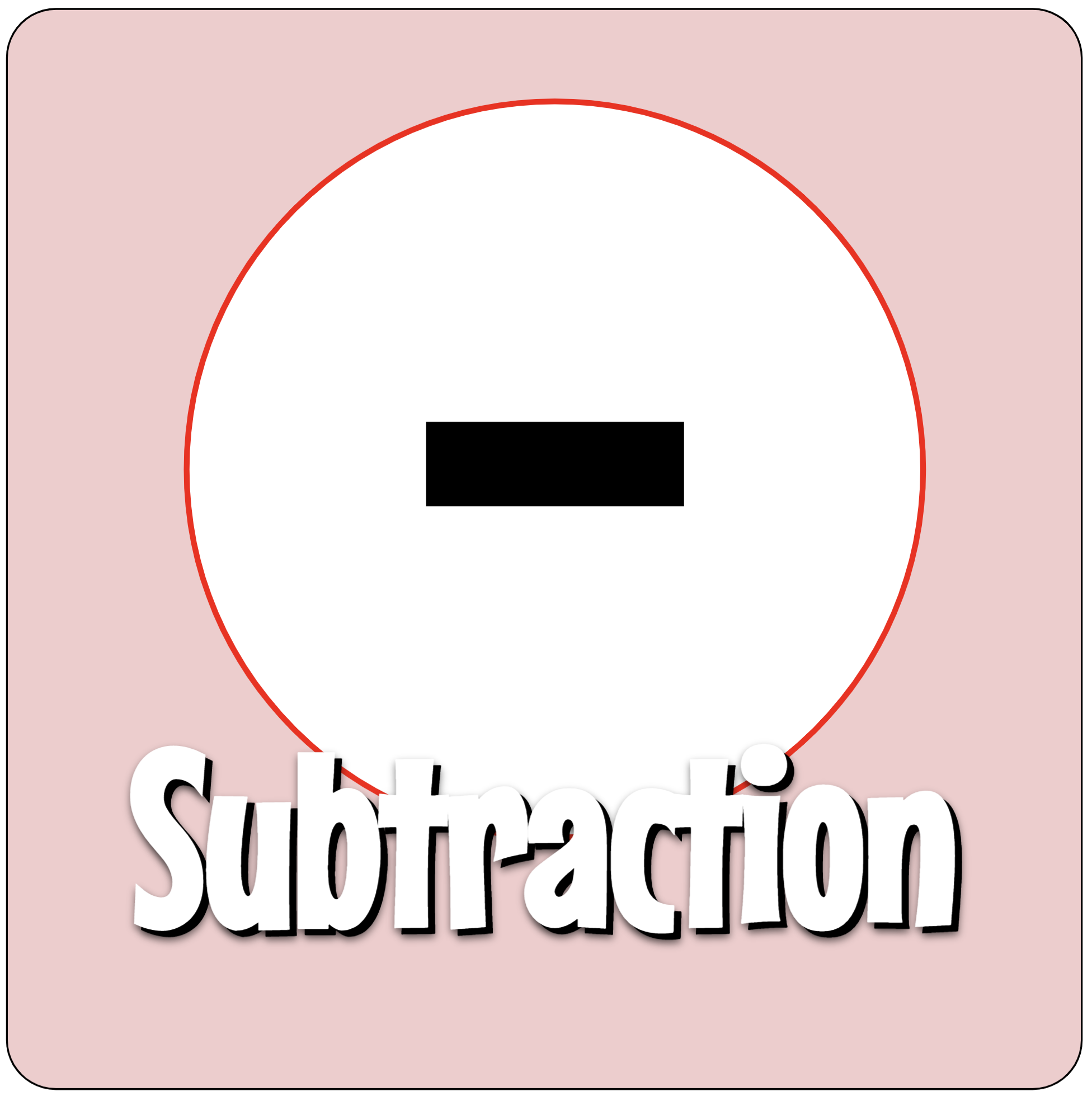Back to Mr. Anker Tests

Back to Math ComputationUse these practice activities to help you with subtraction of whole numbers.
Teachers & Parents: It is recommended that students complete and master each activity before moving on to the next.

 Subtraction 1Subtract from numbers less than 10. Subtraction 2Subtract from numbers less than 10. Subtraction 3Subtract from numbers less than 20. Subtraction 4Subtract from numbers less than 100.
 Subtraction 5- Subtraction 6- Subtraction 7- Subtraction 8-